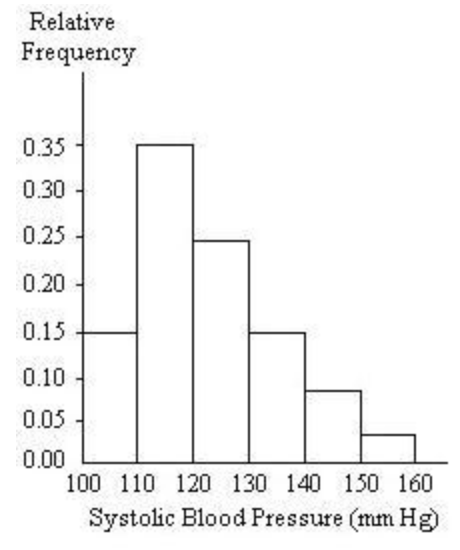##### Question

In: Statistics and Probability

# A nurse measured the blood pressure of each person who visited her clinic.

A nurse measured the blood pressure of each person who visited her clinic. Following is a relative-frequency histogram for the systolic blood pressure readings for those people aged between 25 and 40. The blood pressure readings were given to the nearest whole number. Approximately what percentage of the people aged 25-40 had a systolic blood pressure reading between 110 and 119 inclusive?## Solutions

##### Expert Solution

Concepts and reason

Frequency distribution: In order to carry the data analysis the original data must be grouped in the form of the summary table. This table is called as the frequency distribution table. Histogram: A histogram is a graphical representation of the numerical data. It is a graph that summarizes the distribution of a quantitative variable. Bar heights are used to represent the number of observational units in a particular interval of values. Histogram represents the shape of the distribution of the data that is it shows whether the data is symmetric, right skewed and left skewed distribution. Total frequency: Total frequency is the sum of all frequencies of the class intervals in the frequency distribution table. Relative frequency: Relative frequency is obtained by dividing the respective frequencies of each class by the total number of observations in the study.

Fundamentals

Formula of total frequency is,

$$\begin{array}{c} \text { Total }= \\ \qquad f_{1}+f_{2}+\ldots+f_{n} \\ =\sum_{i=1}^{n} f_{i} \end{array}$$

Where, $$f_{1}, f_{2}, \ldots, f_{n}$$ are the class frequencies of the 1 st, 2 nd and nth interval. Formula for relative frequency distribution is, Relativefrequency $$=\frac{\text { Classfrequency }}{\text { Totalfrequency }}$$

Formula for the percentage of the observations lies in specified class is, Percentage $$=$$ RelativeFrequencyofspecifiedclass $$\times 100$$

The objective of the problem is obtained below:

From the given information, relative frequency histogram is constructed for the systolic blood pressure readings for those people aged between 25 and $$40 .$$ The percentage of the people aged $$25-40$$ had a systolic blood pressure reading between 110 and 119 inclusive is obtained by using Percentage $$=$$ Relative frequency of specified class $$\times 100$$.

The main objective of the problem is to find the percentage of the people aged $$25-40$$ had a systolic blood pressure reading between 110 and 119 inclusive.

The percentage of the people aged $$25-40$$ had a systolic blood pressure reading between 110 and 119 inclusive is obtained below:

From the histogram, the relative frequency of the people aged $$25-40$$ had a systolic blood pressure reading between 110 and 119 is $$0.35 .$$ Percentage $$=$$ Relative frequency of specified class $$\times 100$$

$$\begin{array}{c} =0.35 \times 100 \\ =35 \% \end{array}$$

Thus, the percentage of the people aged $$25-40$$ had a systolic blood pressure reading between 110 and 119 inclusive is $$35 \%$$

The percentage of the people aged $$25-40$$ had a systolic blood pressure reading between 110 and 119 inclusive is obtained by multiplying the relative frequency of that class by 100 .

Thus, the percentage of the people aged $$25-40$$ had a systolic blood pressure reading between 110 and 119 inclusive is $$35 \%$$